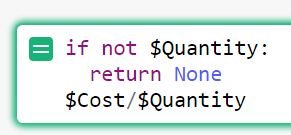# #TypeError - How to resolve errors in a formula column due to empty cells

Formulas apply to all rows within a column - so what happens when rows don’t have data? You’ll get an error, as you can see below. In the “Cost per item” column, we are using a formula = `\$Cost/\$Quantity` but there is no data in rows some of these rows, so we see `#TypeError`.

We can change a column’s behavior on different rows using a conditional in the formula. For the example above, we’ll want to add the `if` statement, seen below, before the formula - that way, when `\$Quantity` is blank, the cell will be blank and the rest of the logic will only apply when `\$Quantity` is not blank.

``````if not \$Quantity:
return None
``````

Our final formula entry will look like this;Please note that to add a new line within the formula entry box, select [SHIFT] + [ENTER]. Be sure to follow the format above, replacing `Quantity` with the name of your data column, and don’t forget to add the colon after the if statement and indent the return statement.

After updating the formula, we no longer have #TypeErrors where no quantity is entered.

More examples can be found at the links below

Intro to Formulas - Varying Formula By Row

October 2020 Newsletter - Quick Tips: Conditional Expressions

3 Likes